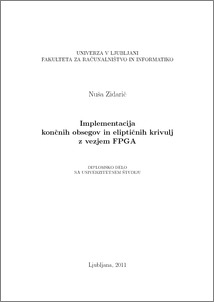# Implementation of finite fields and elliptic curves on a FPGA device

Nuša Zidarič (2011) Implementation of finite fields and elliptic curves on a FPGA device. EngD thesis.Preview
PDF

## Abstract

Nowadays, elliptic curve cryptosystems are widely distributed. Its fundamental operation is scalar multiplication kP, where P is a point of the elliptic curve and k an integer. Following the need for fast scalar multiplication, we decided for a hardware implementation on a FPGA device to achieve an adequate speed-up and increase in throughput. Hardware implementation is additionally simplified by the use of XOR gates for polynomial addition performed in GF(2m). Squaring turned out to be quite simple and low-cost as well. As one of the most common operations in finite field arithmetic, efficiently implemented multiplication can significantly improve performance of the entire design. Best results were achieved by using the hybrid Montgomery multiplier that was able to compute the product in just two clock cycles. Exponentiation was implemented by the square and multiply algorithm, where the use of a combinatiorial multiplication circuit gave the biggest gain in performance. The best method for inversion proved to be the extended Euclidean algorithm. Exponentiation and inversion turned out to be relatively time consuming, since they both require a high number of iterations. Performance of elliptic curve arithmetic is dependent upon efficiency of binary field operations. The usage of projective coordinates significantly improves point addition and point doubling; it allows us to use only multiplications and squarings and to avoid inversion up to the last moment, i.e. the conversion of the projective point back to ordinary coordinates. Best results were achieved using projective coordinates Lopez-Dahab, which also prooved to be the best choice for point multiplication, that was implemented by a series of point additions and point doublings.

Item Type: Thesis (EngD thesis)
Keywords: FPGA, hardware implementation, binary finite fields, Montgomery multiplication, extended Euclidean algorithm, elliptic curves, projective coordinates
Number of Pages: 123
Language of Content: Slovenian
Mentor / Comentors:
Name and SurnameIDFunction
prof. dr. Aleksandar Jurišić1118Mentor
doc. dr. Patricio Bulić255Comentor